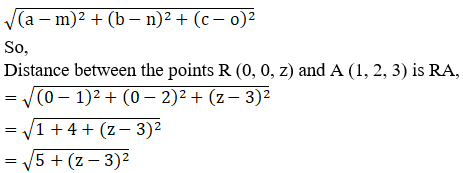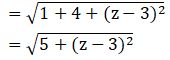Find the points on z-axis which are at a distance $$\sqrt{21}$$ from the point (1, 2, 3).

Asked by Sakshi | 1 year ago |  30

##### Solution :-

Given:

The point (1, 2, 3)

Distance = $$\sqrt{21}$$

We know x = 0 and y = 0 on z-axis

Let R(0, 0, z) any point on z-axis

According to question:

RA = $$\sqrt{21}$$

RA2 = 21

By using the formula,

The distance between any two points (a, b, c) and (m, n, o) is given by,We know, RA2 = 21

5 + (z – 3)2 = 21

z2+ 9 – 6z + 5 = 21

z2 – 6z = 21 – 14

z2– 6z – 7 = 0

z2– 7z + z – 7 = 0

z(z– 7) + 1(z – 7) = 0

(z– 7) (z + 1) = 0

(z– 7) = 0 or (z + 1) = 0

z= 7 or z = -1

The points (0, 0, 7) and (0, 0, -1) on z-axis is equidistant from (1, 2, 3).

Answered by Aaryan | 1 year ago

### Related Questions

#### A(1, 2, 3), B(0, 4, 1), C(-1, -1, -3) are the vertices of a triangle ABC. Find the point in which the bisector of the

A(1, 2, 3), B(0, 4, 1), C(-1, -1, -3) are the vertices of a triangle ABC. Find the point in which the bisector of the angle ∠BAC meets BC.

#### The mid-points of the sides of a triangle ABC are given by (-2, 3, 5), (4, -1, 7) and (6, 5, 3). Find the coordinates

The mid-points of the sides of a triangle ABC are given by (-2, 3, 5), (4, -1, 7) and (6, 5, 3). Find the coordinates of A, B and C.

#### If the points A(3, 2, -4), B(9, 8, -10) and C(5, 4, -6) are collinear, find the ratio in which C divided AB.

If the points A(3, 2, -4), B(9, 8, -10) and C(5, 4, -6) are collinear, find the ratio in which C divided AB.